# Unit-4: Counter and Registers

## Unit-4: Counter and RegistersFlipflop

a flipflop is a binary storage device. It can store binary bit either 0 or 1. it has two stable states high and low i.e. 1 or 0. It has property to remain in state indefinitely until it is directed by input signal to switch over the other state.

The basic formation of flipflop is to store data, they can be used to keep record or what value of variable. Flipflop is also used to exercise control over the functionality of digital circuit i.e. change the operation of a circuit depending on the state of one or more flipflop. Flipflop are mainly used in situation like operation, storage or sequencing.

Flip flop word means that it can be “FLIPPED” into one logic state or “FLOPPED” back into another.

Triggering:

This means making a circuit active. Making a circuit active means allowing the circuit to take input and give output. Like for example supposed we have a flip-flop. When the circuit is not triggered, even if you give some input data, it will not change the data stored inside the flip-flop nor will it change the output Q or Q'. Now there are basically two types of triggering. The triggering is given in form of a clock pulse or gating signal. Depending upon the type of triggering mechanism used, the circuit will become active at specific states of the clock pulse.

1. Level Triggering: In level triggering the circuit will become active when the gating or clock pulse is on a particular level. This level is decided by the designer. We can have a negative level triggering in which the circuit is active when the clock signal is low or a positive level triggering in which the circuit is active when the clock signal is high.
2. Edge Triggering: In edge triggering the circuit becomes active at negative or positive edge of the clock signal. For example if the circuit is positive edge triggered, it will take input at exactly the time in which the clock signal goes from low to high. Similarly input is taken at exactly the time in which the clock signal goes from high to low in negative edge triggering. But keep in mind after the the input, it can be processed in all the time till the next input is taken.

Edge -Triggered Flipflop:

• A Flip Flop that tends to change its state at either a positive edge (rising edge) or negative edge (falling edge) of the clock applied.
• In edge triggering, the clock changes the state of the Flip flop only during the transitions (either positive or negative) of clock pulse. Other parts of the clock has no effect. This is done to increase the noise immunity of the flip flop since the FF is vulnerable to changes by the transients/noise occurring during this period.

SR Flip-Flop

SR flip-flop operates with only positive clock transitions or negative clock transitions.

The block diagram of SR flipflop is shown in figure below.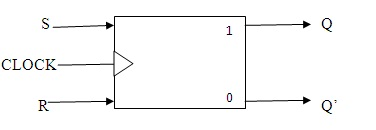The circuit diagram of SR flip-flop is shown in the following figure.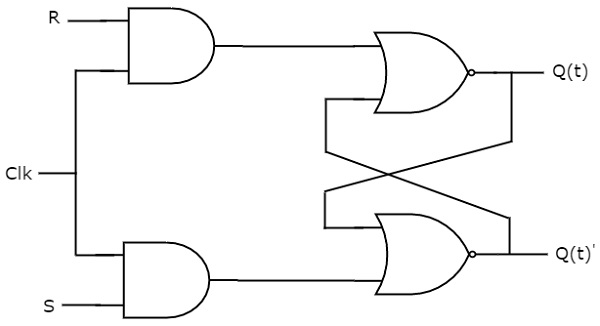This circuit has two inputs S & R and two outputs Qt & Qt’. The operation of SR flipflop is similar to SR Latch. But this flip-flop affects the outputs only when positive transition of the clock signal is applied instead of active enable.

Truth Table: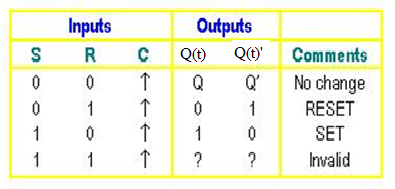Working: -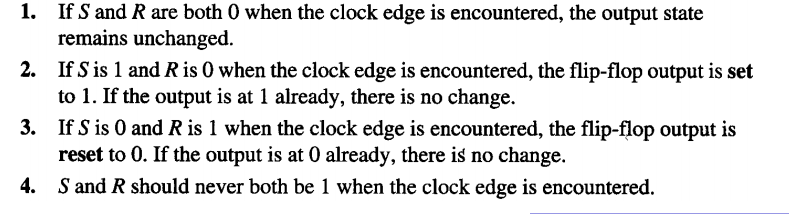The state table of SR flip-flop.

 S R Qt+1 0 0 Qt 0 1 0 1 0 1 1 1 -

Here, Qt & Qt+1 are present state & next state respectively. So, SR flip-flop can be used for one of these three functions such as Hold, Reset & Set based on the input conditions, when positive transition of clock signal is applied.

The characteristic table of SR flip-flop.

 Present Inputs Present State Next State S R Qt Qt+1 0 0 0 0 0 0 1 1 0 1 0 0 0 1 1 0 1 0 0 1 1 0 1 1 1 1 0 x 1 1 1 x

Excitation table

an excitation table shows the minimum inputs that are necessary to generate a particular next state when the current state is known. They are similar to truth tables and state tables, but rearrange the data so that the current state and next state are next to each other on the left-hand side of the table, and the inputs needed to make that state change happen are shown on the right side of the table.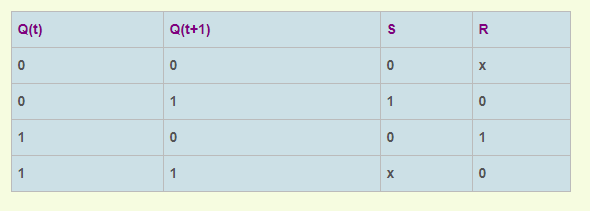D Flip-Flop

D flip-flop operates with only positive clock transitions or negative clock transitions. That means, the output of D flip-flop is insensitive to the changes in the input, D except for active transition of the clock signal. The block diagram of D flip flop is shown in figure below.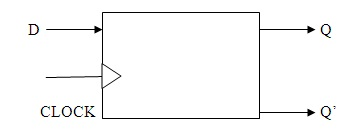The circuit diagram of D flip-flop is shown in the following figure.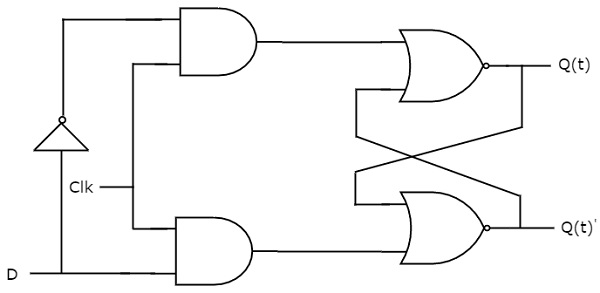This circuit has single input D and two outputs Qt & Qt’. The operation of D flip-flop is similar to D Latch. But this flip-flop affects the outputs only when positive transition of the clock signal is applied instead of active enable.

The truth table of D flipflop is shown below.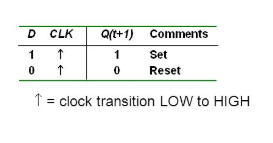Working:

1. When D is high , Q output goes high  on triggering edge of the clock pulse , and flipflop is set.
2. When D is low , The Q output  goes low on the triggering edge of the clock pulse and flip flop is set.

The following table shows the state table of D flip-flop.

 D Qt + 1 0 0 1 1

Therefore, D flip-flop always Hold the information, which is available on data input, D of earlier positive transition of clock signal. From the above state table, we can directly write the next state equation as

Qt+1= D

Next state of D flip-flop is always equal to data input, D for every positive transition of the clock signal. Hence, D flip-flops can be used in registers, shift registers and some of the counters.

Excitation table: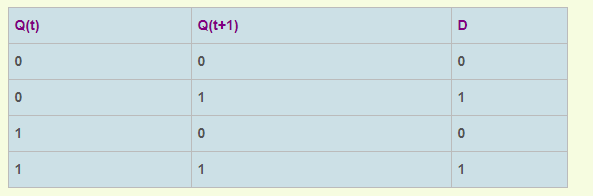JK Flip-Flop

JK flip-flop is the modified version of SR flip-flop. It operates with only positive clock transitions or negative clock transitions.  It is considered to be a universal flip-flop circuit. The sequential operation of the JK Flip Flop is same as for the RS flip-flop with the same SET and RESET input. The difference is that the JK Flip Flop does not the invalid input states of the RS Latch (when S and R are both 1).The block diagram of JK flipflop is shown in figure below.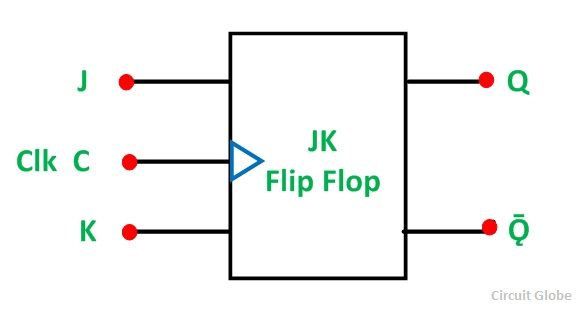The circuit diagram of JK flip-flop is shown in the following figure.

A JK flip-flop has two inputs similar to that of RS flip-flop. A JK flip-flop is nothing but a RS flip-flop along with two AND gates which are augmented to it.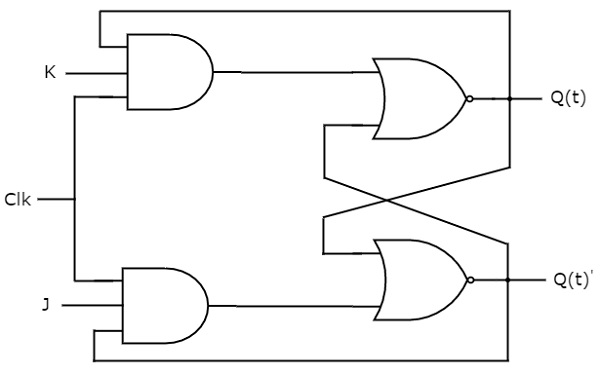This circuit has two inputs J & K and two outputs Qt & Qt’. The operation of JK flip-flop is similar to SR flip-flop.

Truth table of JK flip flop: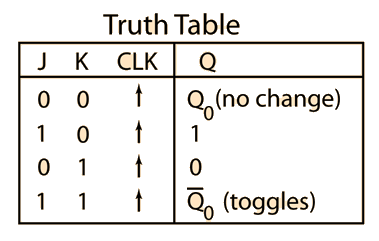Working: -

When J=K=0

When both J and K are 0, the clock pulse has no effect on the output and the output of the flip-flop is the same as its previous value. This is because when both the J and K are 0, the output of their respective AND gate becomes 0.

When J=0, K=1

When J=0, the output of the AND gate corresponding to J becomes 0 (i.e.) S=0 and R=1. Therefore Q’ becomes 0. This condition will reset the flip-flop. This represents the RESET state of Flip-flop.

When J=1, K=0

In this case, the AND gate corresponding to K becomes 0(i.e.) S=1 and R=0. Therefore Q becomes 0. This condition will set the Flip-flop. This represents the SET state of Flip-flop.

When J=K=1

Consider the condition of CP=1 and J=K=1. This will cause the output to complement again and again. This complement operation continues until the Clock pulse goes back to 0. Since this condition is undesirable, we have to find a way to eliminate this condition. This undesirable behavior can be eliminated by Edge triggering of JK flip-flop or by using master slave JK Flip-flops.

The following table shows the state table of JK flip-flop.

 J K Qt+1 0 0 Qt 0 1 0 1 0 1 1 1 Qt'

Here, Qt & Qt+1 are present state & next state respectively. So, JK flip-flop can be used for one of these four functions such as Hold, Reset, Set & Complement of present state based on the input conditions, when positive transition of clock signal is applied.

The following table shows the characteristic table of JK flip-flop.

 Present Inputs Present State Next State J K Qt Qt+1 0 0 0 0 0 0 1 1 0 1 0 0 0 1 1 0 1 0 0 1 1 0 1 1 1 1 0 1 1 1 1 0

Excitation table: -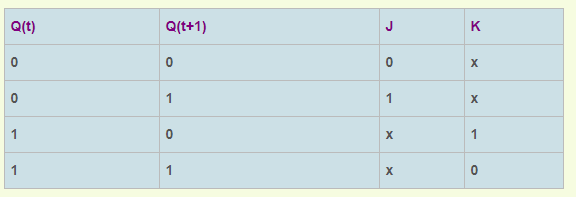T Flip-Flop

T flip-flop is the simplified version of JK flip-flop. It is obtained by connecting the same input ‘T’ to both inputs of JK flip-flop. It operates with only positive clock transitions or negative clock transitions. The block diagram of T flipflop is shown in figure below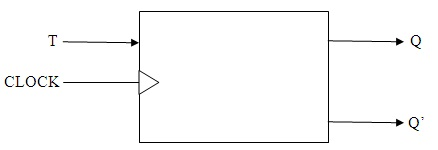This flip-flop has only one input along with Clock pulse. These flip-flops are called T flip-flops because of their ability to complement its state (i.e.) Toggle. So they are called as Toggle flip-flop.

The circuit diagram of T flip-flop is shown in the following figure.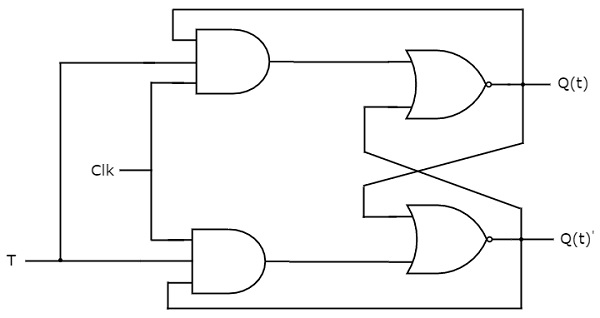This circuit has single input T and two outputs Qt & Qt’. The operation of T flip-flop is same as that of JK flip-flop. Here, we considered the inputs of JK flip-flop as J = T and K = T in order to utilize the modified JK flip-flop for 2 combinations of inputs. So, we eliminated the other two combinations of J & K, for which those two values are complement to each other in T flip-flop.

The following table shows the state table of T flip-flop.

 T Qt+1 0 Qt 1 Qt’

Here, Qt & Qt+1  are present state & next state respectively. So, T flip-flop can be used for one of these two functions such as Hold, & Complement of present state based on the input conditions, when positive transition of clock signal is applied.

Working: -

When T=1 and clock=1, the flip-flop complements its output, regardless of the present state of the Flip-flop. In this case the next state is the complement of the present state.

When T=0, there is no change in the state of the flip-flop (i.e.) the next state is same as the present state of the flip-flop

The following table shows the characteristic table of T flip-flop.

 Inputs Present State Next State T Qt Qt+1 0 0 0 0 1 1 1 0 1 1 1 0

From the above characteristic table, we can directly write the next state equation as

Q(t+1)=T′Q(t)+TQ(t)′

Q(t+1)=TQ(t)

The output of T flip-flop always toggles for every positive transition of the clock signal, when input T remains at logic High 1. Hence, T flip-flop can be used in counters.

Excitation table: -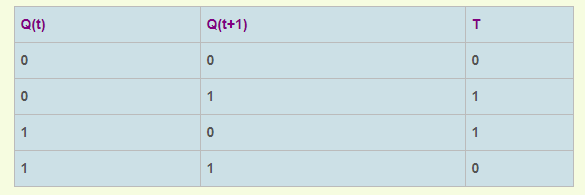Master-Slave JK Flip Flop

Race Around Condition in JK Flip-flop – For J-K flip-flop, if J=K=1, and if clk=1 for a long period of time, then Q output will toggle as long as CLK is high, which makes the output of the flip-flop unstable or uncertain. This problem is called race around condition in J-K flip-flop. This problem (Race Around Condition) can be avoided by ensuring that the clock input is at logic “1” only for a very short time. This introduced the concept of Master Slave JK flip flop.

Master Slave flip flop are the cascaded combination of two flip-flops among which the first is designated as master flip-flop while the next is called slave flip-flop as shown in figure below.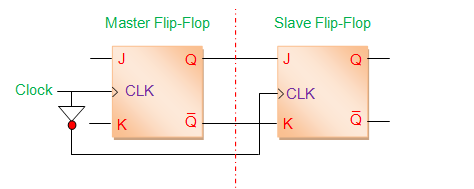Here the master flip-flop is triggered by the external clock pulse train while the slave is activated at its inversion i.e. if the master is positive edge-triggered, then the slave is negative-edge triggered and vice-versa. This means that the data enters into the flip-flop at leading/trailing edge of the clock pulse while it is obtained at the output pins during trailing/leading edge of the clock pulse. Hence a master-slave flip-flop completes its operation only after the appearance of one full clock pulse for which they are also known as pulse-triggered flip-flops.

The internal structure of a master-slave JK flip-flop in terms of NAND gates and an inverter (to complement the clock signal) is shown in Figure below.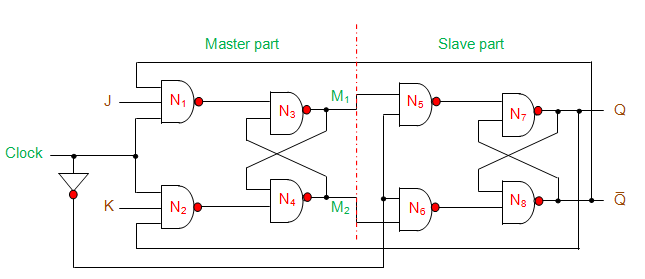Fig:-Master-Slave  JK flip Flop realised using NAND gates and an inverter

Here it is seen that the NAND gate 1 (N1) has three inputs viz., external clock pulse (Clock), input J and output Q̅; while the NAND gate 2 (N2) has external clock pulse (Clock), input K and output Q as its inputs.

Further the outputs of N1 and N2 gates are connected as the inputs for the criss-cross connected gates N3 and N4. These four gates together (N1, N2, N3 and N4) form the master-part of the flip-flop while a similar arrangement of the other four gates N5, N6, N7 and N8 form the slave-part of it.

From figure it is also evident that the slave is driven by the outputs of the master (M1 and M2), which is in accordance with its name master-slave flip-flop. Further the master is active during the positive edge of the clock due to which M1 and M2 change their states; depending on the values of J and K. However, at this instant the outputs of the overall system (master-slave JK flip-flop) remains unchanged as the slave will be inactive due to positive-edge of the clock pulse. Similar to this, the slave decides on its outputs Q and Q̅ depending on its inputs M1 and M2, during the negative edge of the clock during which the master will be inactive.

Truth table

The truth table corresponding to the working of the flip-flop shown in Figure below by Table I.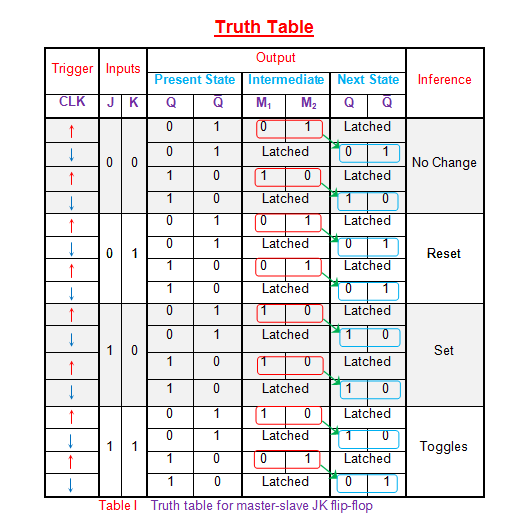Here it is seen that the outputs at the master-part of the flip-flop (data enclosed in red boxes) appear during the positive-edge of the clock (red arrow). However, at this instant the slave-outputs remain latched or unchanged. The same data is transferred to the output pins of the master-slave flip-flop (data enclosed in blue boxes) by the slave during the negative edge of the clock pulse (blue arrow). The same principle is further emphasized in the timing diagram of master-slave flip-flop shown in Figure-3 below.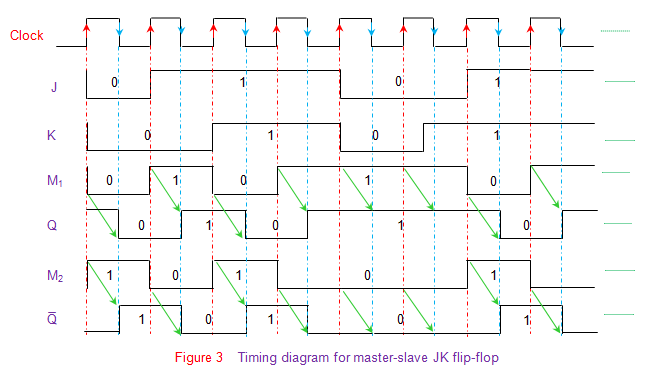Here the green arrows are used to indicate that the slave-output is nothing but the master-output delayed by half-a-clock cycle.

Asynchronous and Synchronous counter

Ripple Counter

Ring Counter

Modulus 10 counter

Modulus counter 5

Modulus counter7

Modulus counter 11

Synchronous design of

Modulus counter 5

Modulus counter7

Modulus counter 11

Application of Counters

• Digital Watch
• Frequency Counter

Latches

Edge-Triggered Flip-Flops

Flip-Flop Operating Characteristics

Flip-Flop Application